Showing first {{hits.length}} results of {{hits_total}} for {{searchQueryText}}{{hits.length}} results for {{searchQueryText}}

Ingen søkeresultater

Author: Cody Herring (May 2013) Procedural graphics in LaTeX: the basics Traditionally, graphics are represented as bitmaps, with anything from 1 bit (black and white) to 24 bits (true color). This allows for simple display of graphics on a traditional (rectangular) computer screen. However, it can be difficult and tedious to generate bitmaps that accurately represent what should be shown on a document or on paper. Procedural graphics help with this by allowing for a more well-defined and easily understood system such as Scalable Vector Graphics (SVG) to be used. By defining vectors, lines, and shapes rather than individual pixels, images can easily be generated for multiple sizes, aspect ratios, pixel densities, printing qualities, and more. This tutorial will provide a brief introduction into procedural graphics using Portable Graphics Format and TikZ: Portable Graphics Format (PGF) is a basic toolset, allowing for simple graphics and figures to be produced; TikZ is an extension to PGF, which allows use of multitude of pre-built figures. Extensive documentation on TikZ and PGF can be found in the PGF Manual. Using PGF and TikZ In order to use the TikZ package, it must be imported: \usepackage{tikz} In order to draw pictures, the tikzpicture environment must be used: \begin{tikzpicture} commands go here \end{tikzpicture} Basic drawing TikZ allows for many different shapes and paths to be drawn. Here some basic drawing techniques will be shown. These techniques can be combined to form more complex drawings. Straight paths A straight path from one point to another can be drawn with the \draw command according to an X/Y (horizontal/vertical) coordinate system, like so: \begin{tikzpicture} \draw (5,0) -- (-5, 0); \end{tikzpicture} Multiple \draw calls can also be strung together. This draws a set of axes from -5 to +5 in the X direction, and -2 to +2 in the Y direction. \begin{tikzpicture} \draw (5,0) -- (-5, 0) (0,2) -- (0,-2); \end{tikzpicture}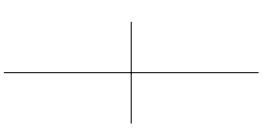Styles can be applied to these lines by adding additional parameters. Here's the same diagram, but with green, dotted, ultra thick lines rotated 15 degrees counterclockwise: \begin{tikzpicture} \draw[green, dotted, ultra thick, rotate=15] (5,0) -- (-5, 0) (0,2) -- (0,-2); \end{tikzpicture}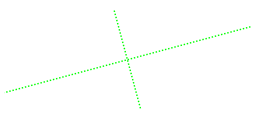Changing the size of a diagram Sometimes the default size of a diagram is too large or too small. Using the scale parameter when declaring the tikzpicture environment allows for different sizes while maintaining the same proportions: \begin{tikzpicture}[scale=0.3] \draw (5,0) -- (-5, 0) (0,2) -- (0,-2); \end{tikzpicture} The following image shows the before and after effect of applying [scale=0.3]: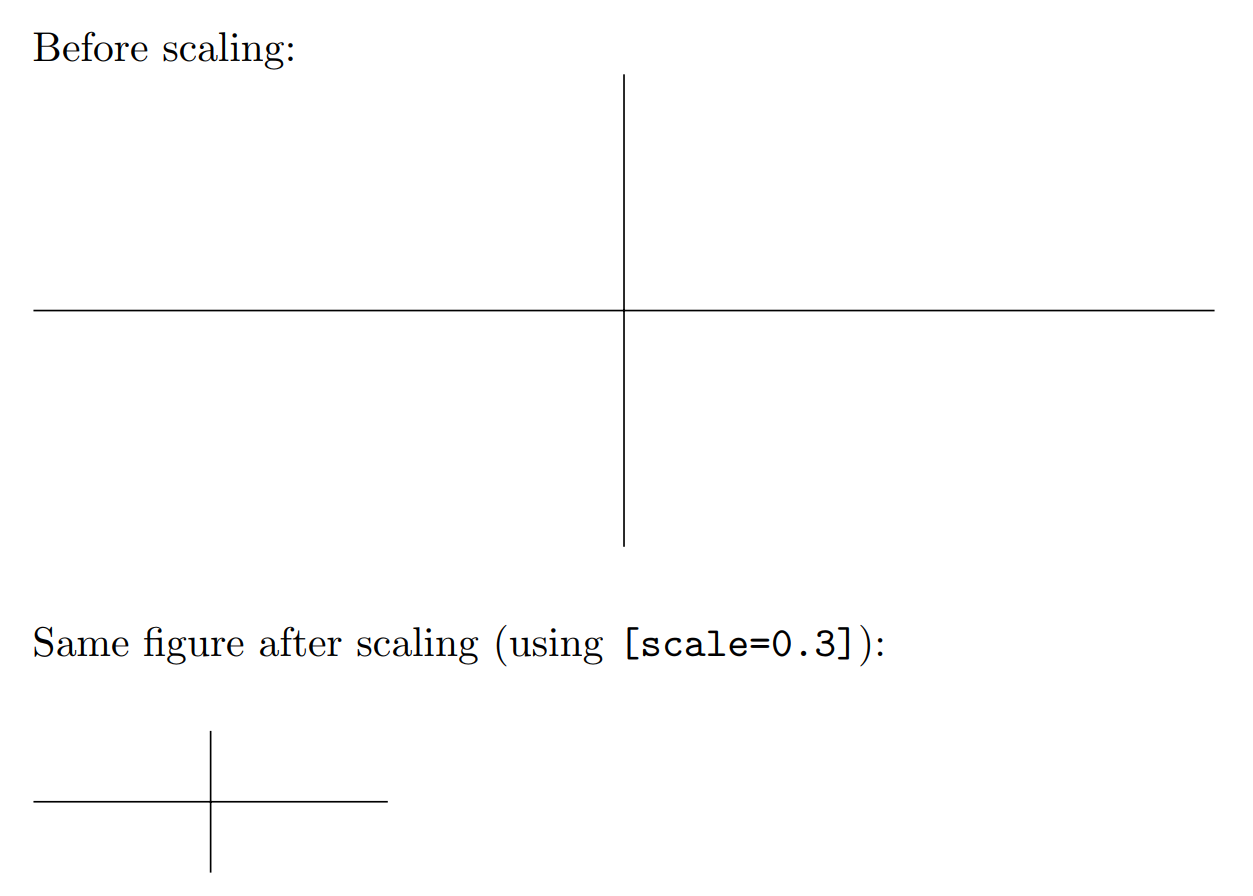Adding arrow tips Arrows can be useful for graphs and diagrams, and they're simple to include in a TikZ picture. The styles demonstrated previously may be used, as well as several different arrow tips. \begin{tikzpicture} \draw[->](0,0) -- (5,0); \end{tikzpicture} \begin{tikzpicture} \draw[<<->>](0,0) -- (5,0); \end{tikzpicture} \begin{tikzpicture} \draw[<<->>, ultra thick, blue](0,0) -- (5,0); \end{tikzpicture} \begin{tikzpicture} \draw[|->>, thick, red](0,0) -- (5,0); \end{tikzpicture}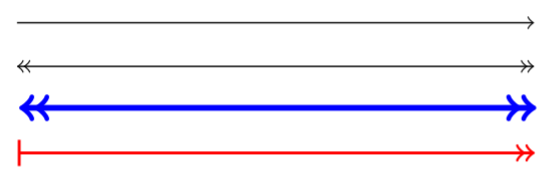Drawing curves The simplest way to draw a curve with TikZ is by specifying the start and end point, and the desired angle leaving the start point and arriving at the end point. \begin{tikzpicture} \draw[ultra thick] (0,0) to [out=75,in=135](3,4); \end{tikzpicture}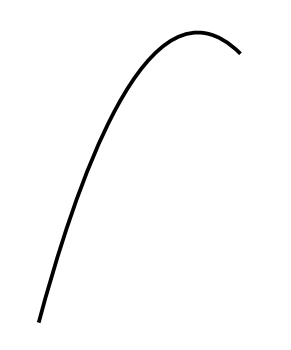Much more complex diagrams can also be created using chained calls. PGF will attempt to draw the smoothest lines possible using the directions provided. \begin{tikzpicture} \draw[ultra thick] (0,0) to [out=90,in=180] (2,2) to [out=270,in=0](0,0) to [out=0,in=90](2,-2) to [out=180,in=270](0,0) to [out=270,in=0](-2,-2) to [out=90,in=180](0,0) to [out=180,in=270](-2,2) to [out=0,in=90](0,0); \end{tikzpicture}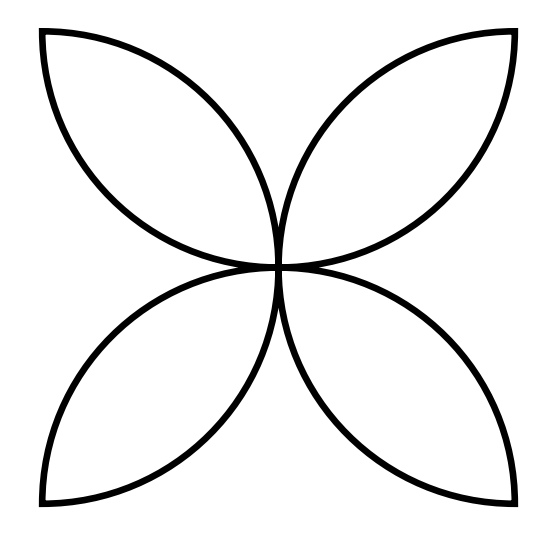Conclusion There's no end to what can be drawn with vector graphics, and their value in allowing for scalable, easily editable diagrams and pictures is a boon to LaTeX users looking for an easy way to use graphics. This post is scratching the surface of what is possible, but should be a good introduction to the capabilities of vector graphics and the TikZ/PGF environment. Additional resources: Minimal introduction to TikZ; CTAN documentation on TikZ & PGF; Overleaf's learn Wiki page.